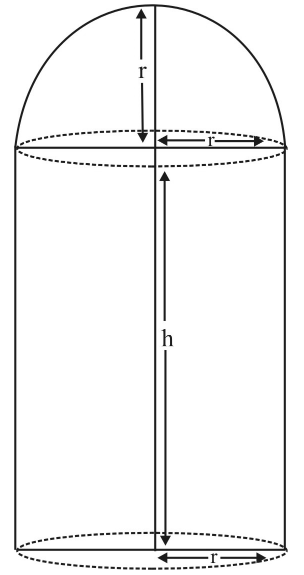# The maximum value of observer’s angle of sight.### Single Variable Calculus: Concepts...

4th Edition
James Stewart
Publisher: Cengage Learning
ISBN: 9781337687805### Single Variable Calculus: Concepts...

4th Edition
James Stewart
Publisher: Cengage Learning
ISBN: 9781337687805

#### Solutions

Chapter 4, Problem 46RE
To determine

## To find: The maximum value of observer’s angle of sight.

Expert Solution

The maximum value of observer’s angle of sight is 30° .

### Explanation of Solution

Given information:

The figure is shown below.

Calculation:

Consider the figure.Figure-1

From figure,

tanα=3x1α=tan1(3x1)tanβ=x1β=tan1(x1)

The sight angle is,

θ=tan1(3x)tan1(x)

Differentiate above equation.

dθdx=11+9x2(3)11+x2

Substitute dθdx=0 in above equation.

0=11+9x2(3)11+x2x=13

Substitute x=13 in above equation.

θ=tan1(33)tan1(13)=π3π6=π6=30°

### Have a homework question?

Subscribe to bartleby learn! Ask subject matter experts 30 homework questions each month. Plus, you’ll have access to millions of step-by-step textbook answers!